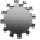Plenary Lecture

Efficient Evaluation of Sparse Jacobians by Matrix CompressionProfessor Andreas Griewank
Department of Mathematics
Humboldt-Universitat zu Berlin
Berlin, Germany
E-mail: griewank@math.hu-berlin.de

Abstract: Many numerical methods in scientific computing require the evaluation of large sparse derivative matrices. It has been known since the seminal work of Curtis Powell and Reid (CPR) that once their sparsity pattern is known Jaconbians can be estimated on the basis of divided differences for a set carefully chosen directions. The number p of such seed directions and thus extra function evaluations can often be limited a priori to a smallish number, which is typically much smaller than the number of independent variables and unaffected by grid sizes and other discretization parameters. The cost factor p is bounded below by the maximal number of nonzeros per row, which is actually sufficient for Jacobian estimation using so-called Newsam-Ramsdell (NR) compression. The NR approach is numerically less stable than the CPR method, which was therefore preferred in practice as divided differences are strongly affected by truncation and round off errors. However now, using automatic or algorithmic differentiation, one obtains directional derivatives with working accuracy and can thus utilize the more economical NR approach. We show how this is done minimizing both the function evaluation costs and the number of arithmetic operations, while ensuring numerical stability.

Brief Biography of the Speaker: http://www.mathematik.hu-berlin.de/~griewank/